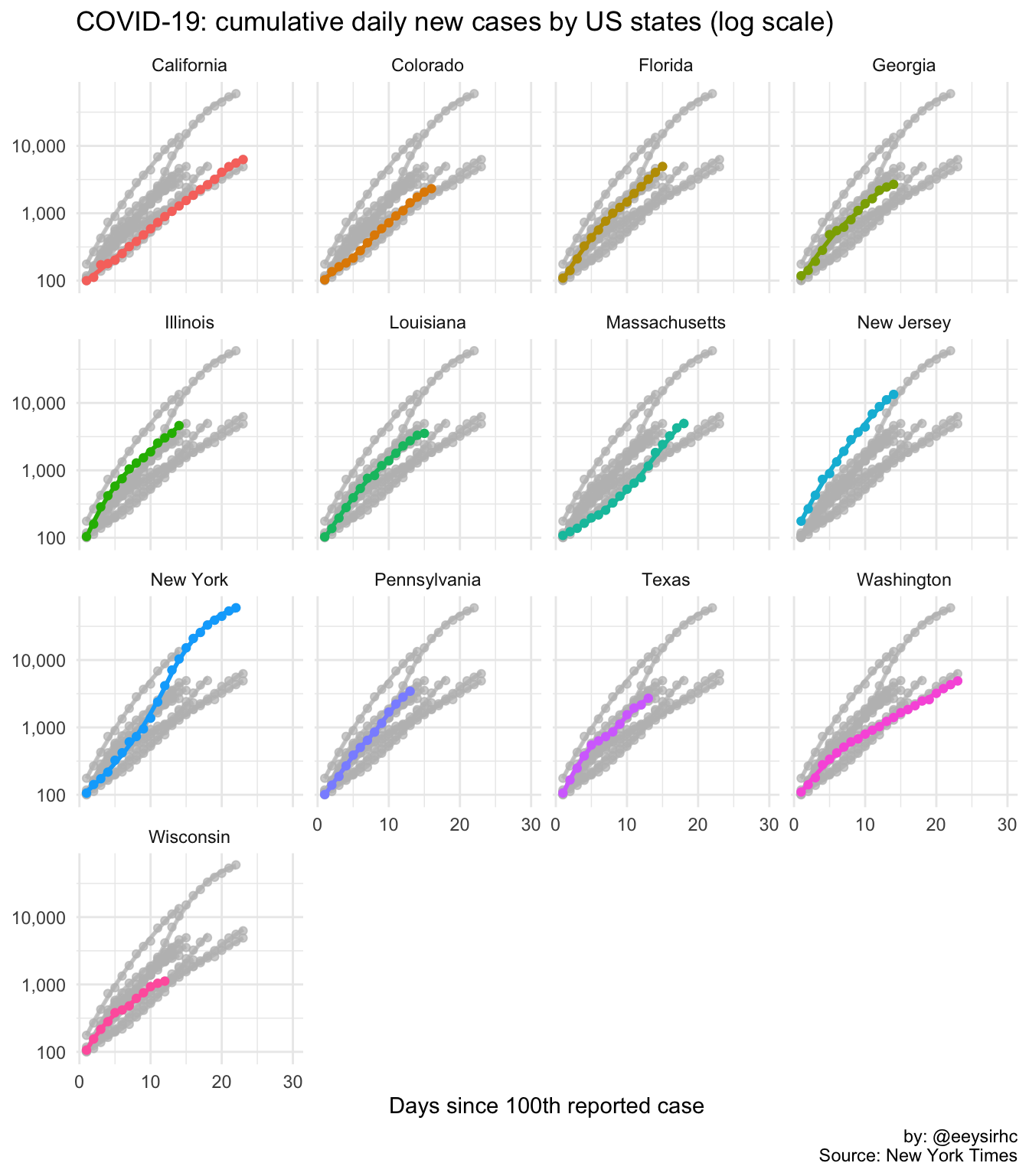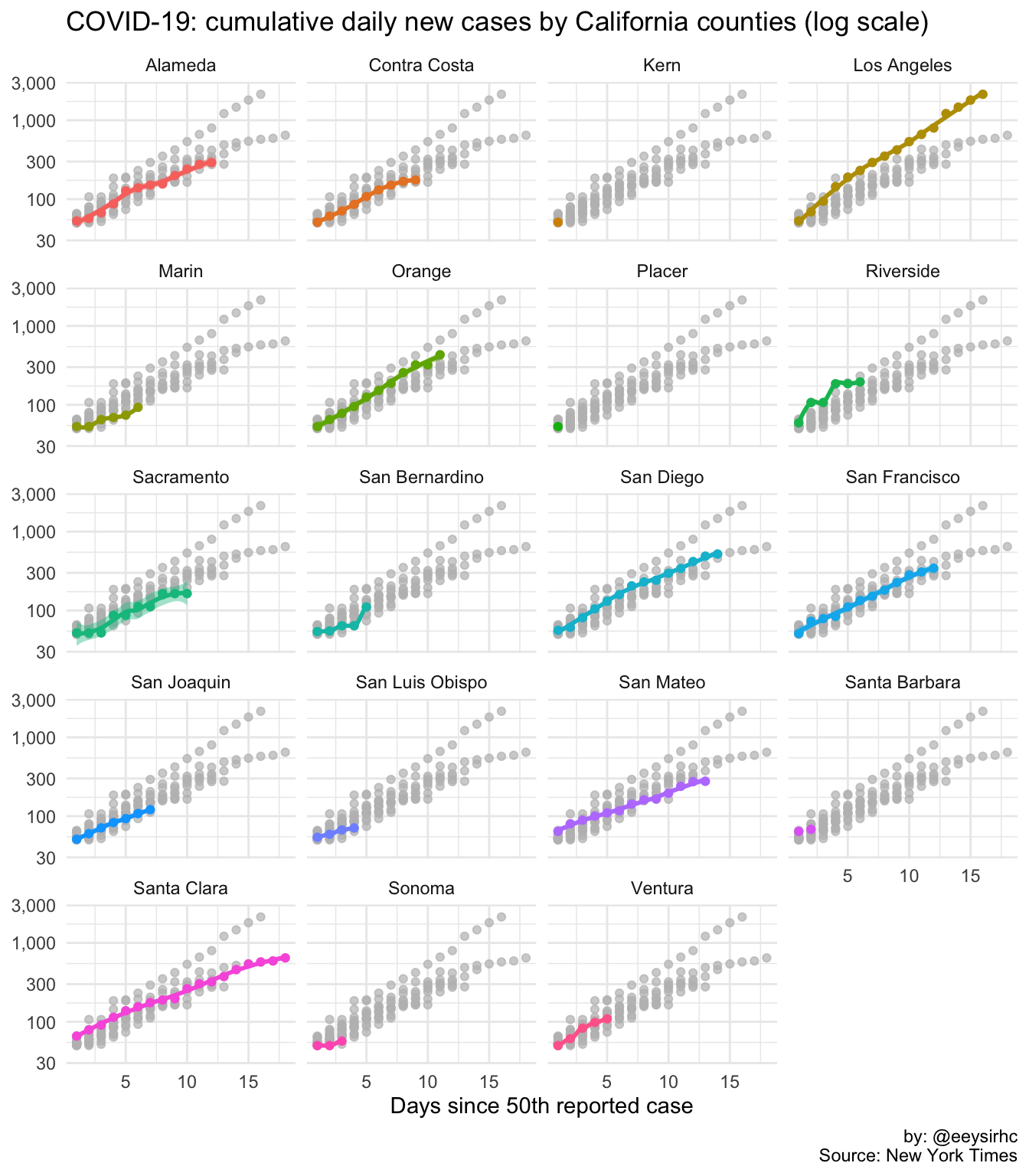A couple weeks ago I shared an #rstats script to track global coronavirus cases by country.

The New York Times also released COVID-19 data for new cases in the United States, both at the state and county level. You can run the code below on a daily basis to get the most up to date figures.

Feel free to modify for your own needs:

``````library(scales)
library(tidyverse)
library(gghighlight)

## State

``````state %>%
group_by(date, state) %>%
mutate(total_cases = cumsum(cases)) %>%
ungroup() %>%
filter(total_cases >= 100) %>% # MINIMUM 100 CASES
group_by(state) %>%
mutate(day_index = row_number(),
n = n()) %>%
ungroup() %>%
filter(n >= 12) %>% # MINIMUM 12 DAYS
ggplot(aes(day_index, total_cases, color = state, fill = state)) +
geom_point() +
geom_smooth() +
gghighlight() +
scale_y_log10(labels = comma_format()) +
facet_wrap(~state, ncol = 4) +
labs(title = "COVID-19: cumulative daily new cases by US states (log scale)",
x = "Days since 100th reported case",
y = NULL, fill = NULL, color = NULL,
caption = "by: @eeysirhc\nSource: New York Times") +
theme_minimal() +
theme(legend.position = 'none') +
expand_limits(x = 30)``````## County

For the county level, we’ll focus only on California:

``````county %>%
filter(state == 'California') %>%
group_by(date, county) %>%
mutate(total_cases = cumsum(cases)) %>%
ungroup() %>%
filter(total_cases >= 50) %>% # MINIMUM 50 CASES
group_by(county) %>%
mutate(day_index = row_number(),
n = n()) %>%
ungroup() %>%
ggplot(aes(day_index, total_cases, color = county, fill = county)) +
geom_point() +
geom_smooth() +
gghighlight() +
scale_y_log10(labels = comma_format()) +
facet_wrap(~county, ncol = 4) +
labs(title = "COVID-19: cumulative daily new cases by California counties (log scale)",
x = "Days since 50th reported case",
y = NULL, fill = NULL, color = NULL,
caption = "by: @eeysirhc\nSource: New York Times") +
theme_minimal() +
theme(legend.position = 'none')``````